# C++ STL的组件

## 容器

 容器 说明 头文件 迭代器 vector vector是创建动态数组的类，该数组允许在后面插入和删除 随机访问 list list是允许从任何位置插入和删除的序列容器。 双向 deque Deque 是 Deque ，允许从两端插入和删除。 随机访问 set set是用于存储唯一集的关联容器。 双向 multiset Multiset是用于存储非唯一集的关联容器。 双向 map Map是用于存储唯一键值对的关联容器，即每个键仅与一个值(一对一映射)相关联。 双向 multimap multimap是用于存储键值对的关联容器，每个键可以与多个值相关联。 双向 stack 后进先出(LIFO)。 没有迭代器 queue 先入先出(FIFO)。 没有迭代器 Priority-queue 第一个元素始终是最高优先级的元素。 没有迭代器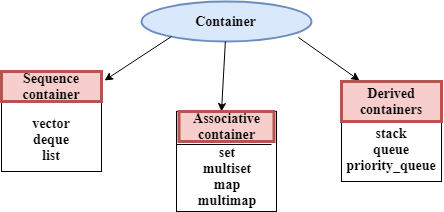## ITERATOR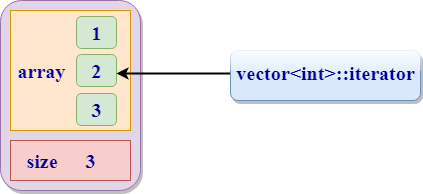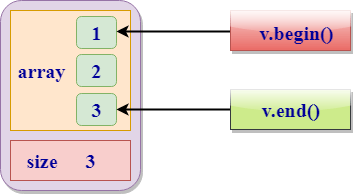## 迭代器类别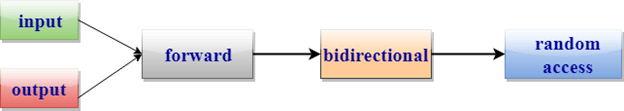迭代器 元素访问 阅读 写 增量操作 比较 输入 -> v = * p ++ ==，!= 输出 * p = v ++ 转发 -> v = * p * p = v ++ ==，!= 双向 -> v = * p * p = v ++，- ==，!= 随机访问 ->，[] v = * p * p = v ++，-，+，-，+ =，-= ==，!=，<，>，<=，> =

## 算法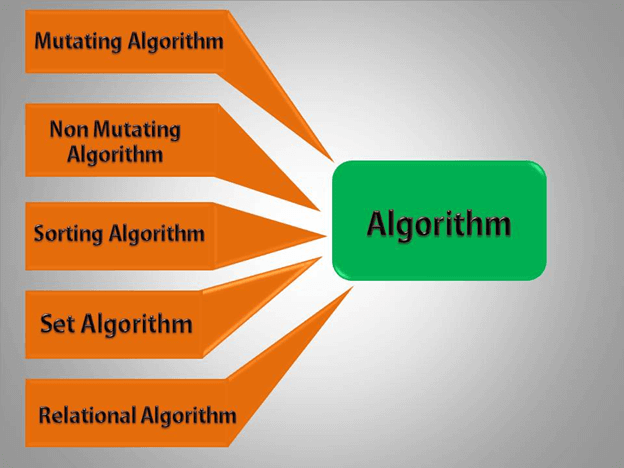## 功能对象

```d();
which is same as:
d.operator() ( );```

```#include <iostream>
using namespace std;
class function_object
{
public:
int operator()(int a, int b)
{
return a+b;
}
};
int main()
{
function_object f;
int result = f(5,5);
cout<<"Addition of a and b is : "<<result;
return 0;
}```

`Addition of a and b is : 10`